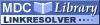Search
Browse
Statistics
Feeds

# Bifurcation analysis of calcium oscillations: time-scale separation, canards, and frequency loweringItem Type: Article Bifurcation analysis of calcium oscillations: time-scale separation, canards, and frequency lowering Schuster, S. and Marhl, M. The behavior of calcium oscillations near bifurcations is analyzed for three different models. For the model developed by Somogyi and Stucki , it is shown that the range of oscillations is bounded by supercritical and subcritical Hopf bifurcations. Near the latter, canard orbits arise, that is, quasi-harmonic oscillations with a very small amplitude grow very fast to become pulsed oscillations. The potential biological significance of this behavior is discussed. A time-scale analysis of this model is performed and an approximation formula for the oscillation period is derived. For two models that we presented earlier [30, 31], it is shown that a homoclinic bifurcation and an infinite period bifurcation, respectively, occur. These imply that the oscillation period can reach arbitrarily high values. This behavior is discussed in the light of frequency encoding, and the scaling laws of the oscillation period are given. Calcium Oscillations, Canard Cycles, Homoclinic Bifurcation, Hopf Bifurcation, Infinite-Period Bifurcation Journal of Biological Systems 0218-3390 World Scientific Publishing 9 4 291-314 1 January 2001 https://doi.org/10.1142/S021833900100044X

Repository Staff Only: item control page

Open Access
MDC Library